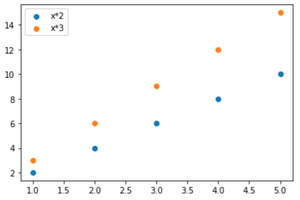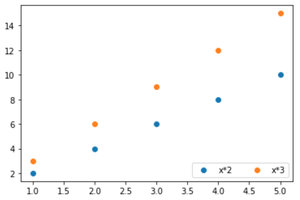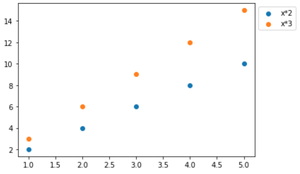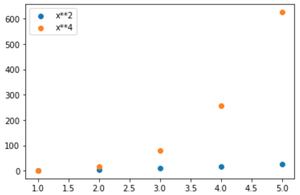Open in App
Not now

# How to add a legend to a scatter plot in Matplotlib ?

• Difficulty Level : Basic
• Last Updated : 10 Nov, 2021

In this article, we are going to add a legend to the depicted images using matplotlib module. We will use the matplotlib.pyplot.legend() method to describe and label the elements of the graph and distinguishing different plots from the same graph.

Syntax: matplotlib.pyplot.legend( [“title_1”, “Title_2”],  ncol = 1 , loc = “upper left” ,bbox_to_anchor =(1, 1) )

Parameters :

• ncol: [takes int, optional parameter] the default value is 1. It represents the number of columns in legend.
• loc: [takes string, optional parameter] the default value is “best” i.e “upper left”. It represents the location of the legend. Other options can be: “best”, “upper right”, “upper left”, “lower left”, “lower right” , “right”, “center left”, “center right”, “lower center”, “upper center”, “center”.
• bbox_to_anchor: [takes a list or tuple of 2 int/float, optional parameter ]. It represents the co-ordinates of legend on the graph. Both x and y co-ordinates are mandatory to give.

Example 1:

## Python3

 `# import required modules` `import` `matplotlib.pyplot as plt`   `# adjust coordinates` `x ``=`  `[``1``,``2``,``3``,``4``,``5``]` `y1 ``=` `[``2``,``4``,``6``,``8``,``10``]` `y2 ``=` `[``3``,``6``,``9``,``12``,``15``]`   `# depict illustration` `plt.scatter(x, y1)` `plt.scatter(x,y2)`   `# apply legend()` `plt.legend([``"x*2"` `, ``"x*3"``])` `plt.show()`

Output:Example 2:

## Python3

 `# impoert required modules` `import` `matplotlib.pyplot as plt`   `# adjust coordinates` `x ``=`  `[``1``,``2``,``3``,``4``,``5``]` `y1 ``=` `[``2``,``4``,``6``,``8``,``10``]` `y2 ``=` `[``3``,``6``,``9``,``12``,``15``]`   `# depict illustration` `plt.scatter(x, y1)` `plt.scatter(x,y2)`   `# apply legend()` `plt.legend([``"x*2"` `, ``"x*3"``], ncol ``=` `2` `, loc ``=` `"lower right"``)` `plt.show()`

Output:Example 3:

## Python3

 `# import required modules` `import` `matplotlib.pyplot as plt`   `# adjust coordinates` `x ``=`  `[``1``,``2``,``3``,``4``,``5``]` `y1 ``=` `[``2``,``4``,``6``,``8``,``10``]` `y2 ``=` `[``3``,``6``,``9``,``12``,``15``]`   `# depict illustration` `plt.scatter(x, y1)` `plt.scatter(x,y2)`   `# apply legend()` `plt.legend([``"x*2"` `, ``"x*3"``], bbox_to_anchor ``=` `(``1` `, ``1``))` `plt.show()`

Output:Note : We may label the elements of the plot in matplotlib.pyplot.scatter() using “label” parameter. However, to show it matplotlib.pyplot.legend() must be written.

Example 4:

## Python3

 `# import required modules` `import` `matplotlib.pyplot as plt` `import` `numpy as np`   `# assign coordinates` `x ``=` `np.arange(``1``, ``6``)` `y1 ``=` `x``*``*``2` `y2 ``=` `x``*``*``4`   `# depict illustration` `plt.scatter(x, y1, label``=``"x**2"``)` `plt.scatter(x, y2, label``=``"x**4"``)`   `# apply legend()` `plt.legend()` `plt.show()`

Output:Note: If matplotlib.pyplot.legend() is not written in code, then labels will not be shown.

My Personal Notes arrow_drop_up
Related Articles